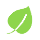## Your Money: When should you sell a bond fund?## Calculate the Macaulay Duration of the fund with prevailing interest rates and then take a call

When investing in a bond or bond fund, typically investors look to hold the bond till maturity while receiving periodic interest. But sometimes investors may need to know the minimum period to hold the bond fund in case they are looking to switch or redeem it, especially in the context of changing interest rates.

The Macaulay duration of a bond or bond fund tells us how long to hold the instrument to protect the principal amount invested.

What is Macaulay Duration
The Macaulay Duration of a bond is the weighted average number of years an investor must hold onto a bond until the present values of the bond’s cash flows equal the bond price paid. The cash flows include the periodic interest or coupon payments, and the maturity amount (if held to maturity) or the sale proceeds (if sold before maturity).

The weight of each cash flow is determined by dividing the present value of the cash flow by the bond price. This weight, when multiplied by the duration of the cash flow, gives us the weighted duration of each cash flow. When summed up, these weighted durations give us the Macaulay Duration of a bond.

In the case of a bond fund, the fund manager invests in different bonds of different terms to maturity so the Macaulay Duration of the fund as a whole satisfies the objectives of the fund. This involves considering the weighted average durations of all the cash flows from all the bonds invested in by the fund.

An illustration
Let us say a bond with a face value of Rs 1,000 and coupon rate of 6% is set to mature in two years with yearly payment of Rs 60. Assume interest rate is also 6%.

Now we want to know how long to hold this investment so that we at least recoup our invested principal amount of Rs 1000. This is given by the Macaulay Duration.

1st cash flow = Rs 60 after 1 year
Present value of 1st cash flow = Rs 60 / ( 1 + 6% ) ^ 1 = Rs 56.6 (where 6% is the interest rate)
Weight of 1st cash flow = Rs 56.6 / Rs 1000 = 0.0566
Weighted duration of 1st cash flow = 0.0566 x 1 year = 0.0566 years

Similarly,
2nd cash flow = Rs 60 after 2 years + Rs 1000 after 2 years = Rs 1060 after 2 years
Present value of 2nd cash flow = Rs 1060 / ( 1 + 6% ) ^ 2 = Rs 943.3
Weight of 2nd cash flow = Rs 943.3 / `1000 = 0.9433
Weighted duration of 2nd cash flow = 0.9433 x 2 years = 1.8866 years
Macaulay Duration = 0.0566 + 1.8866 years = 1.9432 years (~23 months)

How do investors use the Macaulay Duration
One way of reading the above results is that, since the Macaulay Duration is almost the same as the maturity term of the bond, there is no point in selling this bond prematurely. This is also borne out by the fact that the bond’s coupon rate of 6% is equal to the prevailing interest rate of 6% in the market, so there is little benefit in selling before term.

On the other hand, if interest rates in the market increase to 8%, the bond offering 6% coupon rate now looks unattractive.

So, investors may want to redeem their bond investment before maturity and invest in the new opportunity.

In this case, they should first calculate the Macaulay Duration using 8% as the interest rate in the above calculations, which comes to around 22 months. If capital protection is the aim, then the bond should not be sold before 22 months. If reinvesting for better returns is the aim, then the bond should not be held after 22 months. In case of bond funds, the portfolio’s Macaulay Duration needs to be compared with prevailing interest rates.